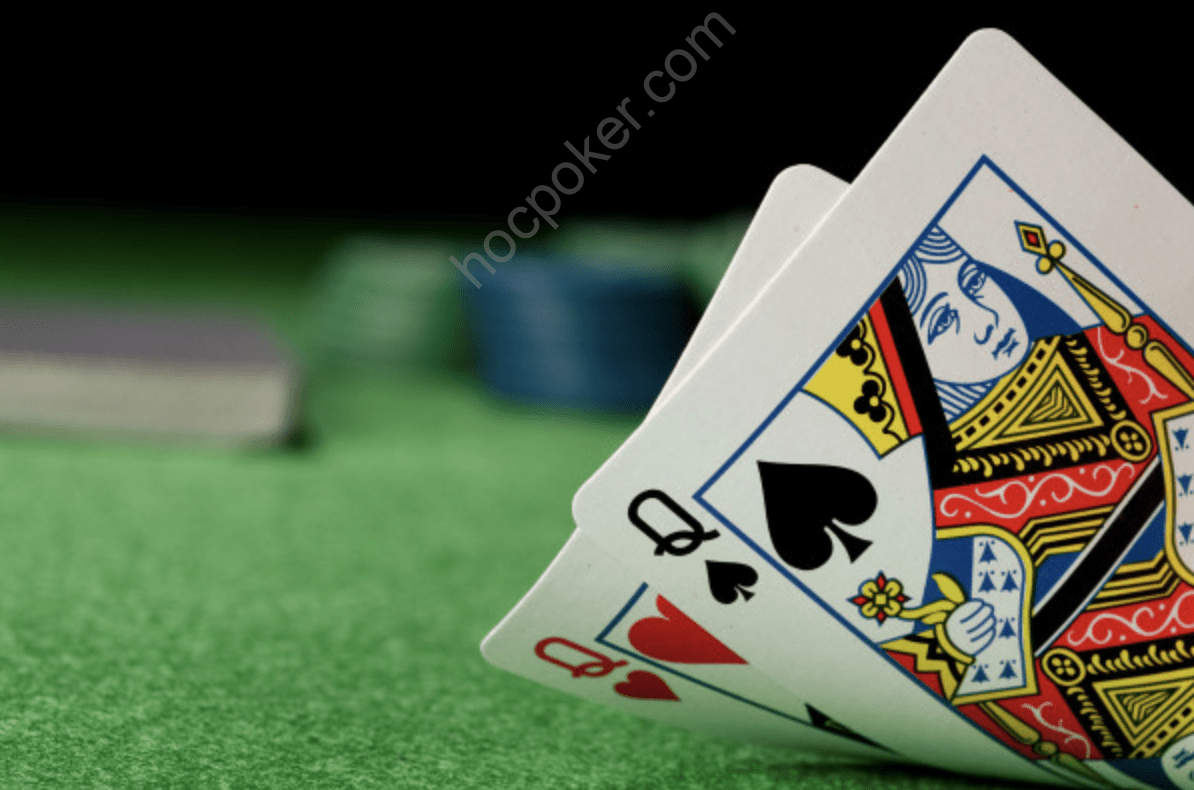# Hand CombinationsAnother important basic component of poker math is hand combi‐ nations, i.e. how many different ways there are to make up a hand. For example, with A‐K the answer is 16 as there are four aces and four kings that can each match up. But if an ace comes on the flop there are now only 12, and so on. Pocket pairs are different since they are two cards of the same rank. If you write out the four differ‐ ent colors for any given card, and draw lines between them repre‐ senting all the combinations, you will see there are six combinations. How about a suited connector? There are four ways to make any given suited connector (one of each suit).

EV Calculations

Now we have covered some of the basics it is time to attempt a sim‐ ple EV calculation to see how the math of poker actually works in practice. For example, consider the following:

(8/46)(\$350 + \$1,000) + (38/46)(–\$350) = –\$54

This is a quick way of saying that 8 out of 46 times one event will happen, and when it does we gain \$350 + \$1,000, but when the other event happens (which is 38 out of 46 times) we are expected to lose 350 – and then that the overall expected value of this situation is ap‐ proximately minus \$54.

This is a representation of when the opponent goes all in on the turn for \$350 more, and the pot is already \$1,000 and we have an open‐ ended straight draw – exactly eight outs that we know are good and no more. One important thing EV calculations show is that poker decisions are close. It might feel like you are making a call for \$350 to win a \$1,350 pot here; however, in actuality the decision is close and whether you call or fold, or win or lose the pot, the expected value of the different plays is nowhere near the pot size.

For instance in the above situation, it is bad to call over the long haul as a call will cost us money and the \$54 loss is relatively large. Play around with the numbers in the equation and you will see that the pot would need to be \$300 bigger to make the call about breakeven and \$600 bigger before there is around a \$50 expected value gain.

Poker is all about these decisions – not making decisions to win \$1,500 pots but making decisions to gain a small amount in expected value.

The idea of an EV calculation is to consider every possible action we can take and figure out which earns us the most expected value. Here the options are to either call or fold. If we fold our expected value is 0 – we can’t lose any more money because we aren’t risking anymore by putting it in the pot and we also cannot gain any money because we are folding so there is no way to win the pot. The hand ends right there and our expected value is 0. So we can then con‐ sider what other options we have and see if they are better than that (i.e. if the expected value is higher than 0). A couple of ways to set up the equation are:

(x/46)(\$350 + \$1,000) + (46 – x)/46)(–\$350) = 0

Here, solving the equation for x, and 46–x (where x is the number of outs we need to have to make our call) will reveal where the break‐ even point is. So if we have that many outs or more then our ex‐ pected value is higher than zero and it’s a better play than folding. Another way to set up the equation is to keep the number of outs the same and change the pot size to see what pot size is required for us to make it a profitable call:

(8/46)(\$350 + x) + (38/46)(–\$350) = 0

And one final way to set it up is to change how much the opponent goes all‐in for on the river in order to see how much that needs to be for it to become a profitable play:

(8/46)(x + \$1,000) + (38/46)(–x) = 0

By taking situations that come up and turning them into EV equa‐ tions you can learn a lot. By playing around with the numbers and equations it will show what the important factors are in a hand, and how much they need to change one way or the other to make plays profitable or unprofitable. It will show when some plays aren’t as important as you thought they were because the EV isn’t much above or below 0, and help you decide whether you have made a good or bad decision in the play of an actual hand, so that you will be aware of it in the future.Previous post Poker MathNext post Putting it all together